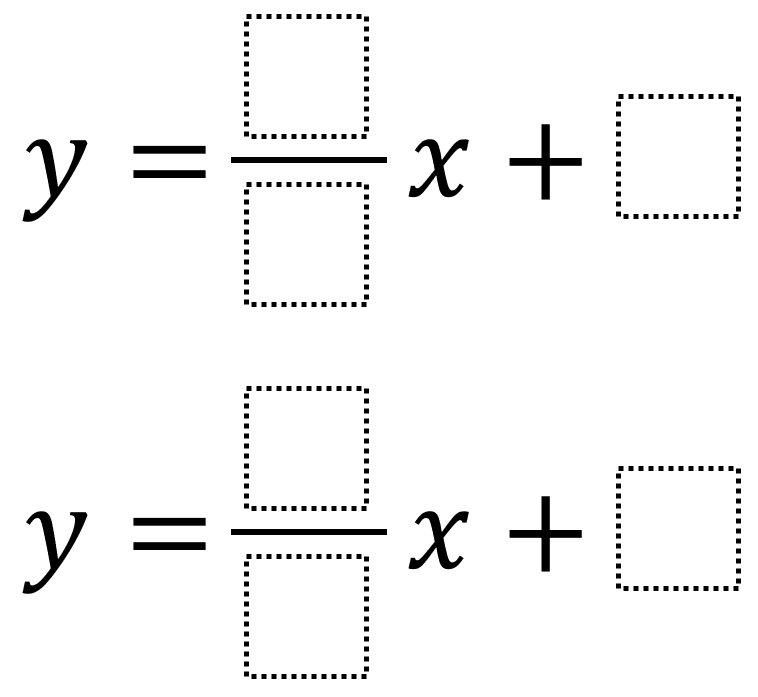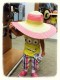# Systems of Equations 2

Directions: Using the integers -9 to 9 at most one time each, place an integer in each box to create a system of equations with a solution in that’s as close to the origin as possible.### Hint

How can you tell which solution is closer to the origin?

The solution that is closest to the origin which has been found so far is y = (9/-1)x + -2 and y = (8/1)x + 2 which has a solution at (-4/17, 2/17). Have you found a solution that is even closer to 0? Let us know in the comments.

Source: Robert Kaplinsky

## Square Root Expression 2

Directions: Using the digits 0 to 9 at most one time each, place a digit …

1.Even closer to zero system is: y=9/8x+0 and y=-9/1x+-1 it hits the point (8/81, 1/9)

2.I stumbled upon this by accident:
y = (9/1)x+(-1) and y = (-4/4)x+0
The intersection is (1/10, -1/10)

••3.